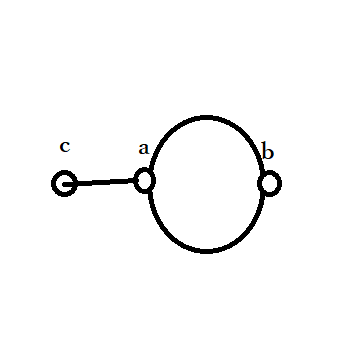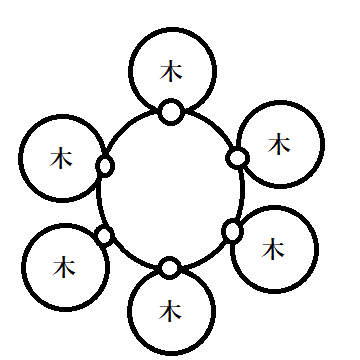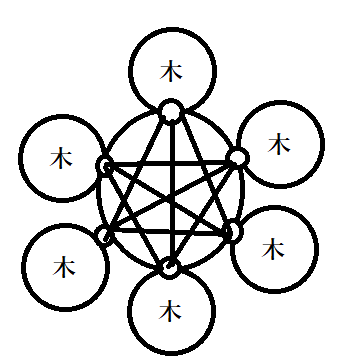# AGC 038 D - Unique Path

Type 0 has transitivity, that is for all a, b, c, if there is exactly one path between a and b and there is exactly one path between b and c, then there is exactly one path between a and c. So type 0 is an equivalence relation.

For any equivalence relation, we can consider equivalence class. In this problem, each equivalence class forms a tree. It is intuitive, isn't it?

On the other hand, type 1 doesn't have transitivity. For example, in the following figure, a => b and b => c doesn't imply a => c.After making trees, connect trees like circle.If there are k trees, at least k edges are required to form a circle, and at most k*(k-1)/2 edges can be used optionally.